# Data structure 6.pptx

Dec. 1, 2022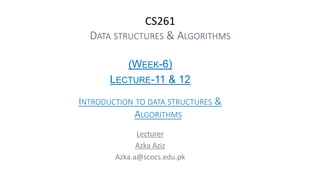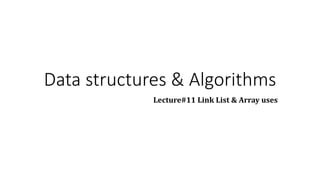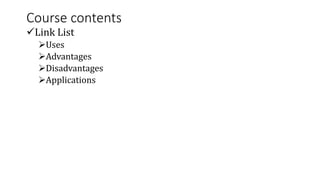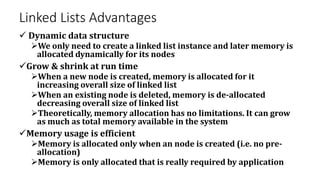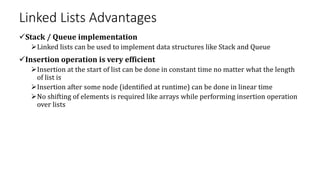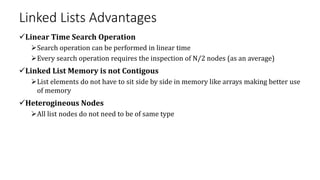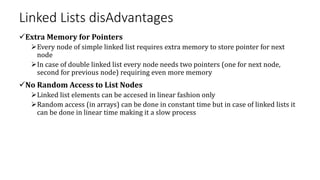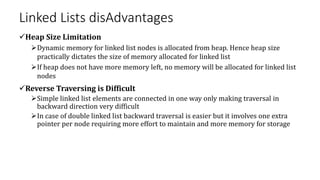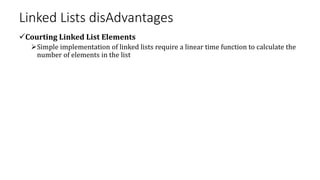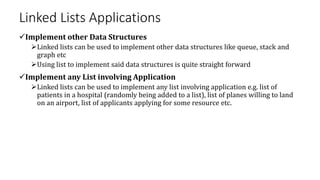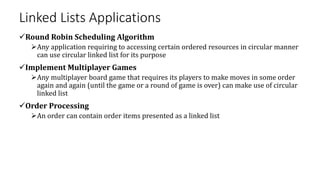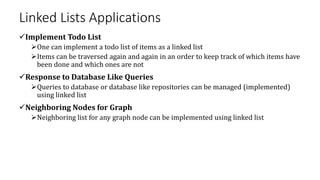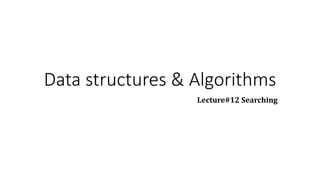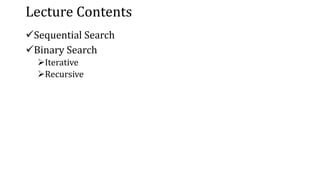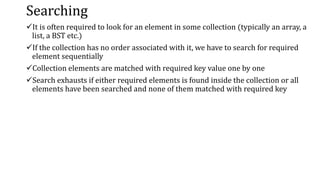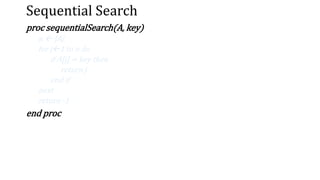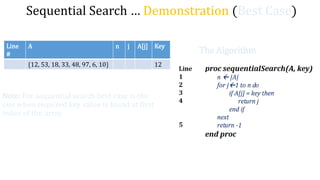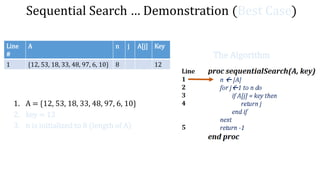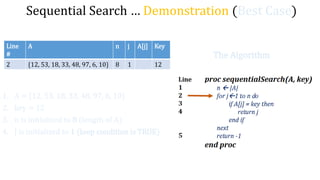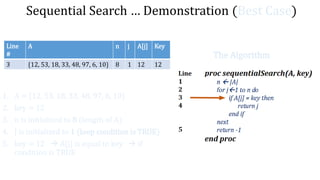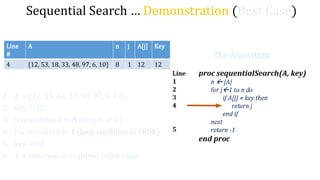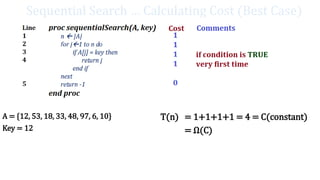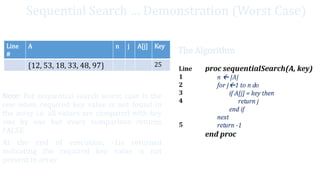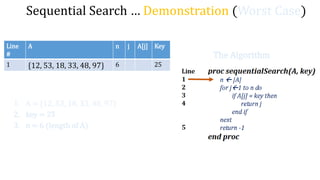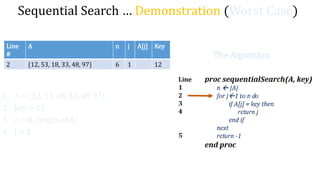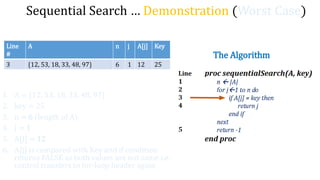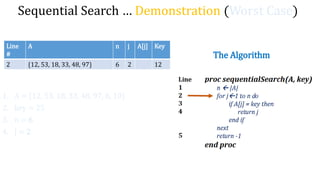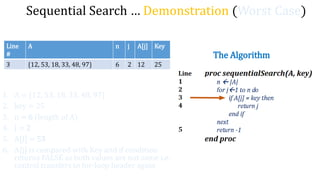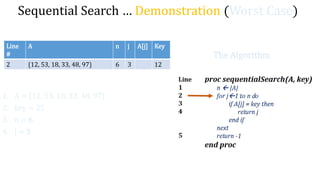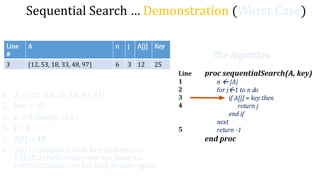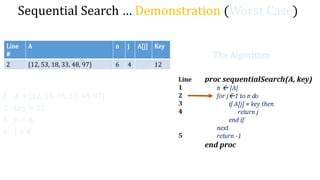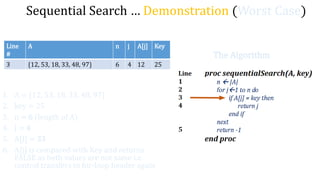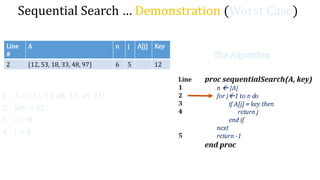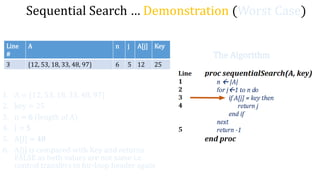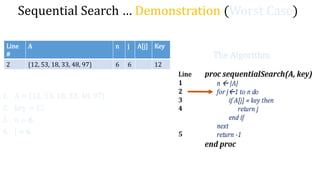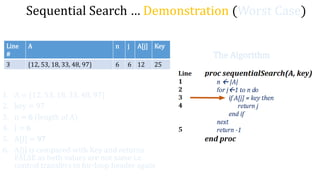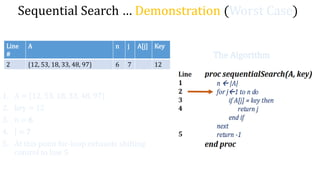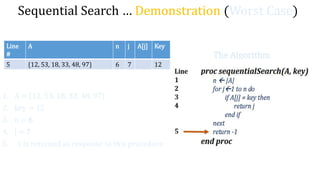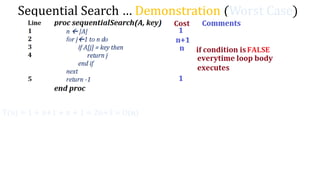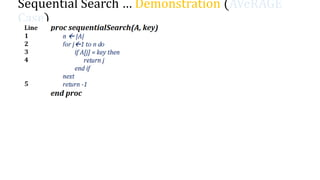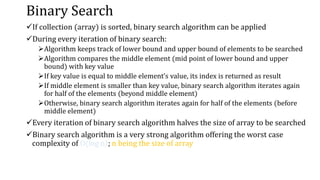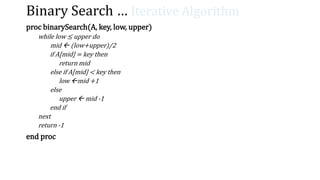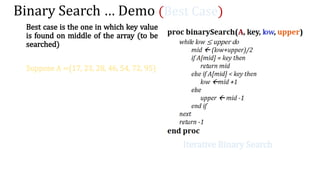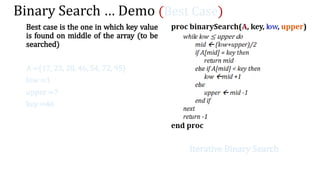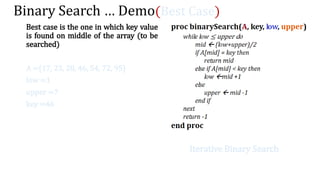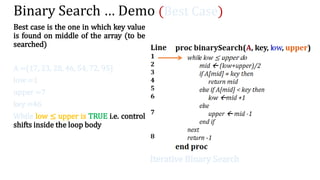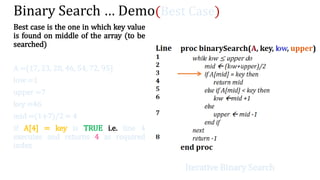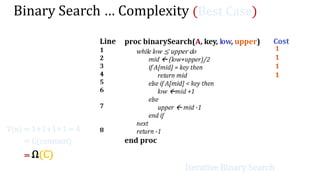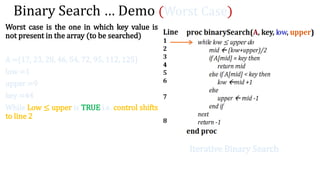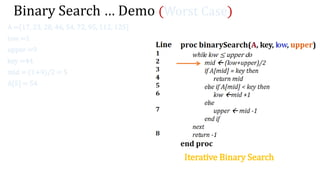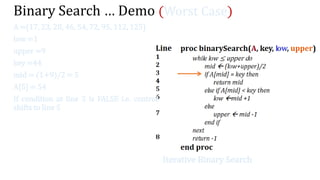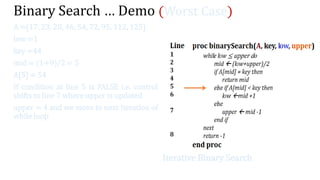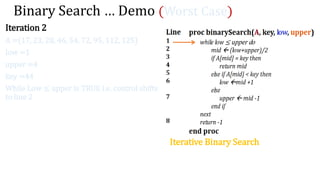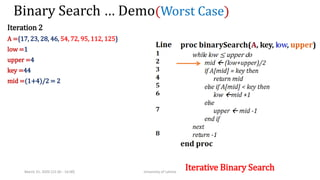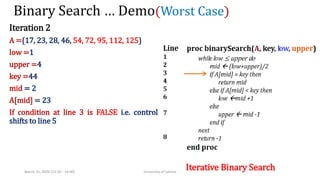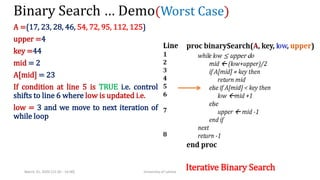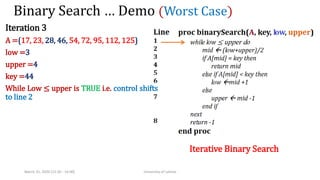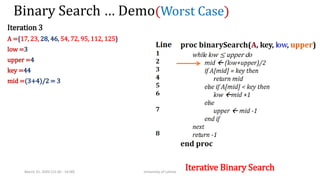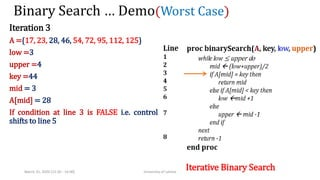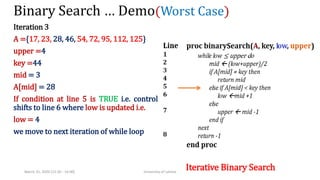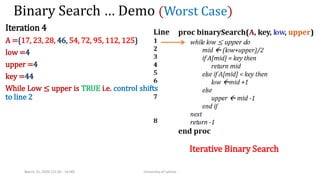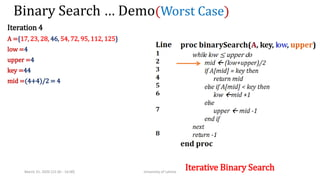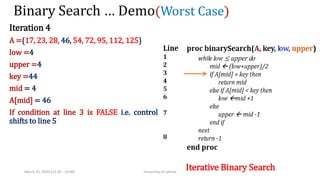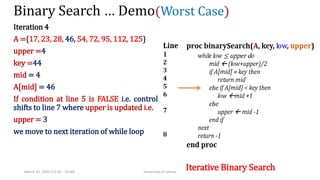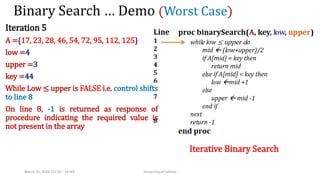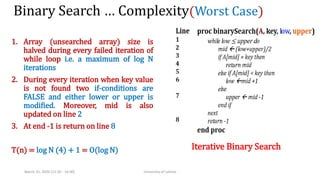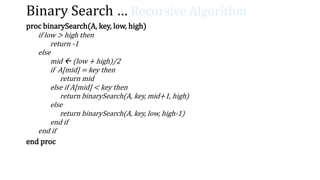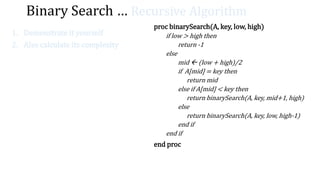1 of 68

### Data structure 6.pptx

• 1. CS261 DATA STRUCTURES & ALGORITHMS (WEEK-6) LECTURE-11 & 12 INTRODUCTION TO DATA STRUCTURES & ALGORITHMS Lecturer Azka Aziz Azka.a@scocs.edu.pk
• 2. Data structures & Algorithms Lecture#11 Link List & Array uses
• 4. Linked Lists Advantages  Dynamic data structure We only need to create a linked list instance and later memory is allocated dynamically for its nodes Grow & shrink at run time When a new node is created, memory is allocated for it increasing overall size of linked list When an existing node is deleted, memory is de-allocated decreasing overall size of linked list Theoretically, memory allocation has no limitations. It can grow as much as total memory available in the system Memory usage is efficient Memory is allocated only when an node is created (i.e. no pre- allocation) Memory is only allocated that is really required by application
• 5. Linked Lists Advantages Stack / Queue implementation Linked lists can be used to implement data structures like Stack and Queue Insertion operation is very efficient Insertion at the start of list can be done in constant time no matter what the length of list is Insertion after some node (identified at runtime) can be done in linear time No shifting of elements is required like arrays while performing insertion operation over lists
• 6. Linked Lists Advantages Linear Time Search Operation Search operation can be performed in linear time Every search operation requires the inspection of N/2 nodes (as an average) Linked List Memory is not Contigous List elements do not have to sit side by side in memory like arrays making better use of memory Heterogineous Nodes All list nodes do not need to be of same type
• 7. Linked Lists disAdvantages Extra Memory for Pointers Every node of simple linked list requires extra memory to store pointer for next node In case of double linked list every node needs two pointers (one for next node, second for previous node) requiring even more memory No Random Access to List Nodes Linked list elements can be accesed in linear fashion only Random access (in arrays) can be done in constant time but in case of linked lists it can be done in linear time making it a slow process
• 8. Linked Lists disAdvantages Heap Size Limitation Dynamic memory for linked list nodes is allocated from heap. Hence heap size practically dictates the size of memory allocated for linked list If heap does not have more memory left, no memory will be allocated for linked list nodes Reverse Traversing is Difficult Simple linked list elements are connected in one way only making traversal in backward direction very difficult In case of double linked list backward traversal is easier but it involves one extra pointer per node requiring more effort to maintain and more memory for storage
• 9. Linked Lists disAdvantages Courting Linked List Elements Simple implementation of linked lists require a linear time function to calculate the number of elements in the list
• 10. Linked Lists Applications Implement other Data Structures Linked lists can be used to implement other data structures like queue, stack and graph etc Using list to implement said data structures is quite straight forward Implement any List involving Application Linked lists can be used to implement any list involving application e.g. list of patients in a hospital (randomly being added to a list), list of planes willing to land on an airport, list of applicants applying for some resource etc.
• 11. Linked Lists Applications Round Robin Scheduling Algorithm Any application requiring to accessing certain ordered resources in circular manner can use circular linked list for its purpose Implement Multiplayer Games Any multiplayer board game that requires its players to make moves in some order again and again (until the game or a round of game is over) can make use of circular linked list Order Processing An order can contain order items presented as a linked list
• 12. Linked Lists Applications Implement Todo List One can implement a todo list of items as a linked list Items can be traversed again and again in an order to keep track of which items have been done and which ones are not Response to Database Like Queries Queries to database or database like repositories can be managed (implemented) using linked list Neighboring Nodes for Graph Neighboring list for any graph node can be implemented using linked list
• 13. Data structures & Algorithms Lecture#12 Searching
• 14. Lecture Contents Sequential Search Binary Search Iterative Recursive
• 15. Searching It is often required to look for an element in some collection (typically an array, a list, a BST etc.) If the collection has no order associated with it, we have to search for required element sequentially Collection elements are matched with required key value one by one Search exhausts if either required elements is found inside the collection or all elements have been searched and none of them matched with required key
• 16. Sequential Search proc sequentialSearch(A, key) n  |A| for j1 to n do if A[j] = key then return j end if next return -1 end proc
• 17. Sequential Search … Demonstration (Best Case) The Algorithm Line # A n j A[j] Key {12, 53, 18, 33, 48, 97, 6, 10} 12 Note: For sequential search best case is the one when required key value is found at first index of the array
• 18. Sequential Search … Demonstration (Best Case) The Algorithm Line # A n j A[j] Key 1 {12, 53, 18, 33, 48, 97, 6, 10} 8 12 1. A = {12, 53, 18, 33, 48, 97, 6, 10} 2. key = 12 3. n is initialized to 8 (length of A)
• 19. Sequential Search … Demonstration (Best Case) The Algorithm Line # A n j A[j] Key 2 {12, 53, 18, 33, 48, 97, 6, 10} 8 1 12 1. A = {12, 53, 18, 33, 48, 97, 6, 10} 2. key = 12 3. n is initialized to 8 (length of A) 4. J is initialized to 1 (loop condition is TRUE)
• 20. Sequential Search … Demonstration (Best Case) The Algorithm Line # A n j A[j] Key 3 {12, 53, 18, 33, 48, 97, 6, 10} 8 1 12 12 1. A = {12, 53, 18, 33, 48, 97, 6, 10} 2. key = 12 3. n is initialized to 8 (length of A) 4. J is initialized to 1 (loop condition is TRUE) 5. key = 12  A[j] is equal to key  if condition is TRUE
• 21. Sequential Search … Demonstration (Best Case) The Algorithm Line # A n j A[j] Key 4 {12, 53, 18, 33, 48, 97, 6, 10} 8 1 12 12 1. A = {12, 53, 18, 33, 48, 97, 6, 10} 2. key = 12 3. n is initialized to 8 (length of A) 4. J is initialized to 1 (loop condition is TRUE) 5. key = 12 6. 1 is returned as required index value
• 22. Sequential Search … Calculating Cost (Best Case) The Algorithm A = {12, 53, 18, 33, 48, 97, 6, 10} Key = 12 T(n) = 1+1+1+1 = 4 = C(constant) = Ω(C)
• 23. Sequential Search … Demonstration (Worst Case) The Algorithm Line # A n j A[j] Key {12, 53, 18, 33, 48, 97} 25 Note: For sequential search worst case is the one when required key value is not found in the array i.e. all values are compared with key one by one but every comparison returns FALSE At the end of execution, -1is returned indicating the required key value is not present in array
• 24. Sequential Search … Demonstration (Worst Case) The Algorithm Line # A n j A[j] Key 1 {12, 53, 18, 33, 48, 97} 6 25 1. A = {12, 53, 18, 33, 48, 97} 2. key = 25 3. n = 6 (length of A)
• 25. Sequential Search … Demonstration (Worst Case) The Algorithm Line # A n j A[j] Key 2 {12, 53, 18, 33, 48, 97} 6 1 12 1. A = {12, 53, 18, 33, 48, 97} 2. key = 25 3. n = 6 (length of A) 4. J = 1
• 26. Sequential Search … Demonstration (Worst Case) The Algorithm Line # A n j A[j] Key 3 {12, 53, 18, 33, 48, 97} 6 1 12 25 1. A = {12, 53, 18, 33, 48, 97} 2. key = 25 3. n = 6 (length of A) 4. J = 1 5. A[J] = 12 6. A[j] is compared with Key and if condition returns FALSE as both values are not same i.e. control transfers to for-loop header again
• 27. Sequential Search … Demonstration (Worst Case) The Algorithm Line # A n j A[j] Key 2 {12, 53, 18, 33, 48, 97} 6 2 12 1. A = {12, 53, 18, 33, 48, 97, 6, 10} 2. key = 25 3. n = 6 4. J = 2
• 28. Sequential Search … Demonstration (Worst Case) The Algorithm Line # A n j A[j] Key 3 {12, 53, 18, 33, 48, 97} 6 2 12 25 1. A = {12, 53, 18, 33, 48, 97} 2. key = 25 3. n = 6 (length of A) 4. J = 2 5. A[J] = 53 6. A[j] is compared with Key and if condition returns FALSE as both values are not same i.e. control transfers to for-loop header again
• 29. Sequential Search … Demonstration (Worst Case) The Algorithm Line # A n j A[j] Key 2 {12, 53, 18, 33, 48, 97} 6 3 12 1. A = {12, 53, 18, 33, 48, 97} 2. key = 25 3. n = 6 4. J = 3
• 30. Sequential Search … Demonstration (Worst Case) The Algorithm Line # A n j A[j] Key 3 {12, 53, 18, 33, 48, 97} 6 3 12 25 1. A = {12, 53, 18, 33, 48, 97} 2. key = 25 3. n = 6 (length of A) 4. J = 3 5. A[J] = 18 6. A[j] is compared with Key and returns FALSE as both values are not same i.e. control transfers to for-loop header again
• 31. Sequential Search … Demonstration (Worst Case) The Algorithm Line # A n j A[j] Key 2 {12, 53, 18, 33, 48, 97} 6 4 12 1. A = {12, 53, 18, 33, 48, 97} 2. key = 25 3. n = 6 4. J = 4
• 32. Sequential Search … Demonstration (Worst Case) The Algorithm Line # A n j A[j] Key 3 {12, 53, 18, 33, 48, 97} 6 4 12 25 1. A = {12, 53, 18, 33, 48, 97} 2. key = 25 3. n = 6 (length of A) 4. J = 4 5. A[J] = 33 6. A[j] is compared with Key and returns FALSE as both values are not same i.e. control transfers to for-loop header again
• 33. Sequential Search … Demonstration (Worst Case) The Algorithm Line # A n j A[j] Key 2 {12, 53, 18, 33, 48, 97} 6 5 12 1. A = {12, 53, 18, 33, 48, 97} 2. key = 12 3. n = 6 4. J = 5
• 34. Sequential Search … Demonstration (Worst Case) The Algorithm Line # A n j A[j] Key 3 {12, 53, 18, 33, 48, 97} 6 5 12 25 1. A = {12, 53, 18, 33, 48, 97} 2. key = 25 3. n = 6 (length of A) 4. J = 5 5. A[J] = 48 6. A[j] is compared with Key and returns FALSE as both values are not same i.e. control transfers to for-loop header again
• 35. Sequential Search … Demonstration (Worst Case) The Algorithm Line # A n j A[j] Key 2 {12, 53, 18, 33, 48, 97} 6 6 12 1. A = {12, 53, 18, 33, 48, 97} 2. key = 12 3. n = 6 4. J = 6
• 36. Sequential Search … Demonstration (Worst Case) The Algorithm Line # A n j A[j] Key 3 {12, 53, 18, 33, 48, 97} 6 6 12 25 1. A = {12, 53, 18, 33, 48, 97} 2. key = 97 3. n = 6 (length of A) 4. J = 6 5. A[J] = 97 6. A[j] is compared with Key and returns FALSE as both values are not same i.e. control transfers to for-loop header again
• 37. Sequential Search … Demonstration (Worst Case) The Algorithm Line # A n j A[j] Key 2 {12, 53, 18, 33, 48, 97} 6 7 12 1. A = {12, 53, 18, 33, 48, 97} 2. key = 12 3. n = 6 4. J = 7 5. At this point for-loop exhausts shifting control to line 5
• 38. Sequential Search … Demonstration (Worst Case) The Algorithm Line # A n j A[j] Key 5 {12, 53, 18, 33, 48, 97} 6 7 12 1. A = {12, 53, 18, 33, 48, 97} 2. key = 12 3. n = 6 4. J = 7 5. -1 is returned as response to this procedure
• 39. Sequential Search … Demonstration (Worst Case) T(n) = 1 + n+1 + n + 1 = 2n+3 = O(n)
• 40. Sequential Search … Demonstration (AVeRAGE Case)
• 41. Binary Search If collection (array) is sorted, binary search algorithm can be applied During every iteration of binary search: Algorithm keeps track of lower bound and upper bound of elements to be searched Algorithm compares the middle element (mid point of lower bound and upper bound) with key value If key value is equal to middle element’s value, its index is returned as result If middle element is smaller than key value, binary search algorithm iterates again for half of the elements (beyond middle element) Otherwise, binary search algorithm iterates again for half of the elements (before middle element) Every iteration of binary search algorithm halves the size of array to be searched Binary search algorithm is a very strong algorithm offering the worst case complexity of O(log n); n being the size of array
• 42. Binary Search … Iterative Algorithm proc binarySearch(A, key, low, upper) while low ≤ upper do mid  (low+upper)/2 if A[mid] = key then return mid else if A[mid] < key then low mid +1 else upper  mid -1 end if next return -1 end proc
• 43. Binary Search … Demo (Best Case) Iterative Binary Search Best case is the one in which key value is found on middle of the array (to be searched) Suppose A ={17, 23, 28, 46, 54, 72, 95}
• 44. Binary Search … Demo (Best Case) Iterative Binary Search Best case is the one in which key value is found on middle of the array (to be searched) A ={17, 23, 28, 46, 54, 72, 95} low =1 upper =7 key =46
• 45. Binary Search … Demo(Best Case) Iterative Binary Search Best case is the one in which key value is found on middle of the array (to be searched) A ={17, 23, 28, 46, 54, 72, 95} low =1 upper =7 key =46
• 46. Binary Search … Demo (Best Case) Iterative Binary Search Best case is the one in which key value is found on middle of the array (to be searched) A ={17, 23, 28, 46, 54, 72, 95} low =1 upper =7 key =46 While low ≤ upper is TRUE i.e. control shifts inside the loop body
• 47. Binary Search … Demo(Best Case) Iterative Binary Search Best case is the one in which key value is found on middle of the array (to be searched) A ={17, 23, 28, 46, 54, 72, 95} low =1 upper =7 key =46 mid =(1+7)/2 = 4 If A = key is TRUE i.e. line 4 executes and returns 4 as required index
• 48. Binary Search … Complexity (Best Case) Iterative Binary Search T(n) = 1+1+1+1 = 4 = C(constant) = Ω(C)
• 49. Binary Search … Demo (Worst Case) Iterative Binary Search Worst case is the one in which key value is not present in the array (to be searched) A ={17, 23, 28, 46, 54, 72, 95, 112, 125} low =1 upper =9 key =44 While Low ≤ upper is TRUE i.e. control shifts to line 2
• 50. Binary Search … Demo (Worst Case) Iterative Binary Search A ={17, 23, 28, 46, 54, 72, 95, 112, 125} low =1 upper =9 key =44 mid = (1+9)/2 = 5 A = 54
• 51. Binary Search … Demo (Worst Case) Iterative Binary Search A ={17, 23, 28, 46, 54, 72, 95, 112, 125} low =1 upper =9 key =44 mid = (1+9)/2 = 5 A = 54 If condition at line 3 is FALSE i.e. control shifts to line 5
• 52. Binary Search … Demo (Worst Case) Iterative Binary Search A ={17, 23, 28, 46, 54, 72, 95, 112, 125} low =1 key =44 mid = (1+9)/2 = 5 A = 54 If condition at line 5 is FALSE i.e. control shifts to line 7 where upper is updated upper = 4 and we move to next iteration of while loop
• 53. Binary Search … Demo (Worst Case) Iterative Binary Search Iteration 2 A ={17, 23, 28, 46, 54, 72, 95, 112, 125} low =1 upper =4 key =44 While Low ≤ upper is TRUE i.e. control shifts to line 2
• 54. Binary Search … Demo(Worst Case) Iterative Binary Search Iteration 2 A ={17, 23, 28, 46, 54, 72, 95, 112, 125} low =1 upper =4 key =44 mid =(1+4)/2 = 2 March 31, 2020 (12:30 - 14:00) University of Lahore
• 55. Binary Search … Demo(Worst Case) Iterative Binary Search Iteration 2 A ={17, 23, 28, 46, 54, 72, 95, 112, 125} low =1 upper =4 key =44 mid = 2 A[mid] = 23 If condition at line 3 is FALSE i.e. control shifts to line 5 March 31, 2020 (12:30 - 14:00) University of Lahore
• 56. Binary Search … Demo(Worst Case) Iterative Binary Search A ={17, 23, 28, 46, 54, 72, 95, 112, 125} upper =4 key =44 mid = 2 A[mid] = 23 If condition at line 5 is TRUE i.e. control shifts to line 6 where low is updated i.e. low = 3 and we move to next iteration of while loop March 31, 2020 (12:30 - 14:00) University of Lahore
• 57. Binary Search … Demo (Worst Case) Iterative Binary Search Iteration 3 A ={17, 23, 28, 46, 54, 72, 95, 112, 125} low =3 upper =4 key =44 While Low ≤ upper is TRUE i.e. control shifts to line 2 March 31, 2020 (12:30 - 14:00) University of Lahore
• 58. Binary Search … Demo(Worst Case) Iterative Binary Search Iteration 3 A ={17, 23, 28, 46, 54, 72, 95, 112, 125} low =3 upper =4 key =44 mid =(3+4)/2 = 3 March 31, 2020 (12:30 - 14:00) University of Lahore
• 59. Binary Search … Demo(Worst Case) Iterative Binary Search Iteration 3 A ={17, 23, 28, 46, 54, 72, 95, 112, 125} low =3 upper =4 key =44 mid = 3 A[mid] = 28 If condition at line 3 is FALSE i.e. control shifts to line 5 March 31, 2020 (12:30 - 14:00) University of Lahore
• 60. Binary Search … Demo(Worst Case) Iterative Binary Search Iteration 3 A ={17, 23, 28, 46, 54, 72, 95, 112, 125} upper =4 key =44 mid = 3 A[mid] = 28 If condition at line 5 is TRUE i.e. control shifts to line 6 where low is updated i.e. low = 4 we move to next iteration of while loop March 31, 2020 (12:30 - 14:00) University of Lahore
• 61. Binary Search … Demo (Worst Case) Iterative Binary Search Iteration 4 A ={17, 23, 28, 46, 54, 72, 95, 112, 125} low =4 upper =4 key =44 While Low ≤ upper is TRUE i.e. control shifts to line 2 March 31, 2020 (12:30 - 14:00) University of Lahore
• 62. Binary Search … Demo(Worst Case) Iterative Binary Search Iteration 4 A ={17, 23, 28, 46, 54, 72, 95, 112, 125} low =4 upper =4 key =44 mid =(4+4)/2 = 4 March 31, 2020 (12:30 - 14:00) University of Lahore
• 63. Binary Search … Demo(Worst Case) Iterative Binary Search Iteration 4 A ={17, 23, 28, 46, 54, 72, 95, 112, 125} low =4 upper =4 key =44 mid = 4 A[mid] = 46 If condition at line 3 is FALSE i.e. control shifts to line 5 March 31, 2020 (12:30 - 14:00) University of Lahore
• 64. Binary Search … Demo(Worst Case) Iterative Binary Search Iteration 4 A ={17, 23, 28, 46, 54, 72, 95, 112, 125} upper =4 key =44 mid = 4 A[mid] = 46 If condition at line 5 is FALSE i.e. control shifts to line 7 where upper is updated i.e. upper = 3 we move to next iteration of while loop March 31, 2020 (12:30 - 14:00) University of Lahore
• 65. Binary Search … Demo (Worst Case) Iterative Binary Search Iteration 5 A ={17, 23, 28, 46, 54, 72, 95, 112, 125} low =4 upper =3 key =44 While Low ≤ upper is FALSE i.e. control shifts to line 8 On line 8, -1 is returned as response of procedure indicating the required value is not present in the array March 31, 2020 (12:30 - 14:00) University of Lahore
• 66. Binary Search … Complexity(Worst Case) Iterative Binary Search 1. Array (unsearched array) size is halved during every failed iteration of while loop i.e. a maximum of log N iterations 2. During every iteration when key value is not found two if-conditions are FALSE and either lower or upper is modified. Moreover, mid is also updated on line 2 3. At end -1 is return on line 8 T(n) = log N (4) + 1 = O(log N) March 31, 2020 (12:30 - 14:00) University of Lahore
• 67. Binary Search … Recursive Algorithm proc binarySearch(A, key, low, high) if low > high then return -1 else mid  (low + high)/2 if A[mid] = key then return mid else if A[mid] < key then return binarySearch(A, key, mid+1, high) else return binarySearch(A, key, low, high-1) end if end if end proc
• 68. Binary Search … Recursive Algorithm proc binarySearch(A, key, low, high) if low > high then return -1 else mid  (low + high)/2 if A[mid] = key then return mid else if A[mid] < key then return binarySearch(A, key, mid+1, high) else return binarySearch(A, key, low, high-1) end if end if end proc 1. Demonstrate it yourself 2. Also calculate its complexity